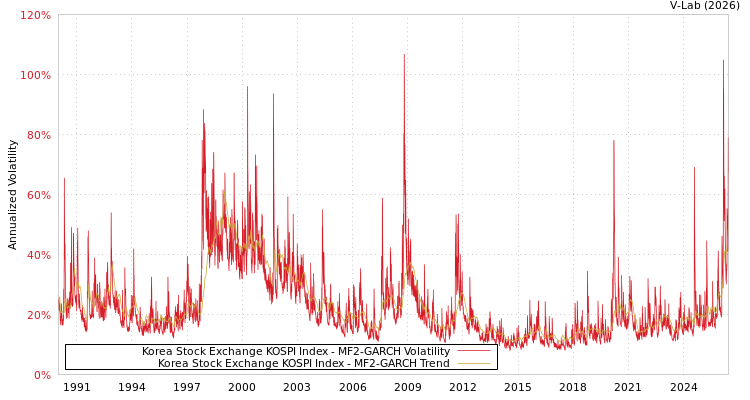##### Korea Stock Exchange KOSPI Index MF2-GARCH Volatility Analysis
###### Volatility Prediction for Monday, June 5th, 2023:11.94% (+0.23%)

Analysis last updated: Friday, June 2, 2023 at 06:34 AM UTC

###### Date Range:

from

to

6M ·

1Y ·

2Y ·

5Y ·

10Y ·

Allparamt-stat
$m$56
$\alpha$0.035313.49
$\beta$0.8388220.55
$\gamma$0.124329.75
${\lambda }_{1}$0.00345.68
${\lambda }_{2}$0.03769.08
${\lambda }_{3}$0.9607217.55
Estimation Period:
Jan 2, 1990 to Jun 2, 2023